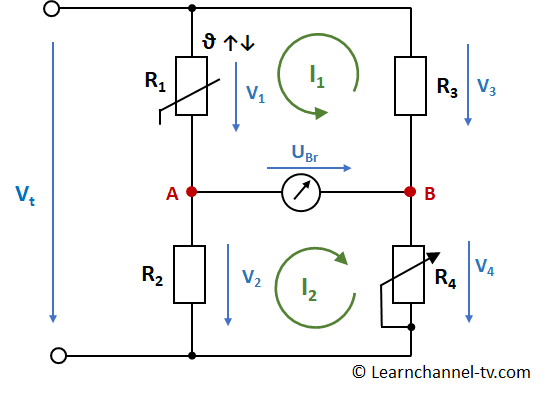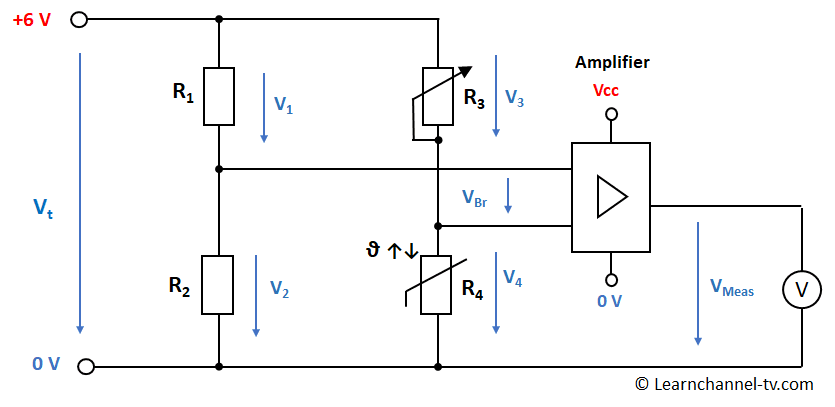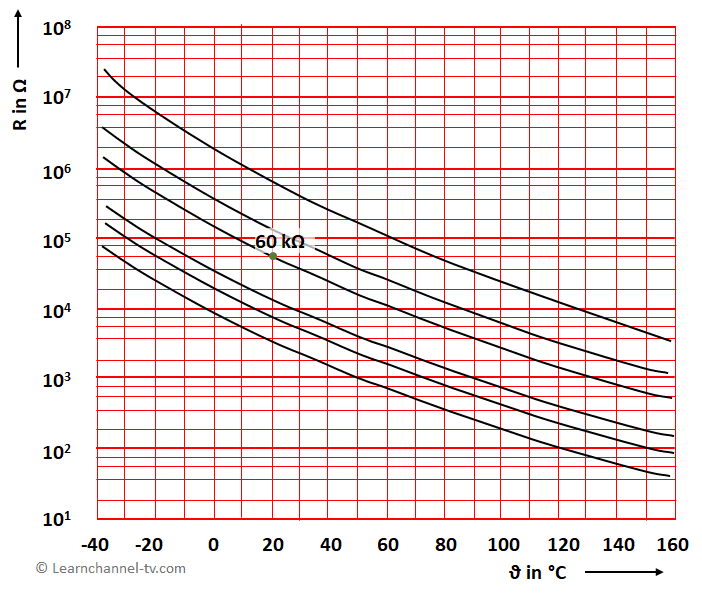# Wheatstone Bridge

## Wheatstone Bridge

### History of this measuring bridge

The so-called measuring bridge has its origins in 1833. At this time, Samuel Hunter Christie (born March 22, 1784 in London; † January 24, 1865), British mathematician and scientist, described the basic principle of the Wheatstone Bridge in a script for the "University of Cambridge". It was also him who proved in 1833 that the conductivity of various metals decreases with increasing length and improved with increasing wire diameter.

However, it was Charles Wheatstone, British physicist (February 6, 1802 in Gloucester, England, † October 19, 1875 in Paris) who recognized the importance and possibilities of this measuring bridge and made this public. Although Charles Wheatstone always named Christie as the inventor of this measuring bridge, this bridge was named after him.

### Function of this measuring bridge:

In our example, a temperature-dependent resistor NTC is installed. As soon as the resistor value changes due to a temperature change, the bridge voltage will also change accordingly. This change in the bridge voltage can be evaluated and thus the actual temperature can be inferred.Wheatstone Bridge

The potentiometer is used to adjust the bridge circuit, if e.g. at a temperature of 0 ° C the bridge voltage should be 0 V. In our example, a temperature-dependent resistor, i.e. NTC, is installed. If the temperature changes, the bridge voltage will also change, which means that the actual temperature can now be inferred.

Why not simply an evaluation via e.g. series connection NTC with resistor?

The advantages of a bridge circuit are as follows:

• A zero adjustment can be carried out so that the bridge voltage can move into the positive or negative.
• Fluctuations in the supply voltage have less of an effect.
• A change can be multiplied by means of a half or full bridge. DMS are often built within a full bridge - more on that later.

Measuring bridge at balance

A measuring bridge is considered to be calibrated, that means at balance, when the bridge voltage UBR = 0 V. This is the case when the resistors R1 and R2 are in the same ratio to R3 and R4:

Adjustment condition VBR = 0 V:

R1   =   R3
R2        R4

Calculate the bridge voltage in the unbalanced state:

Regarding the two loops, there are two possibilities to calculate it:

Via loop l1:        V1 + VBR - V3 = 0 V         =>    VBR = V3 - V1

Alternatively via loop l2:           VBR + V4 - V2= 0 V    =>    VBR = V2 - V4

Voltage drops at individual resistors can be calculated, for example, using the voltage divider formula:

V2   =       R2       *  V
.            R1 + R2               resp.

V4   =     R4          *  V
.            R3 + R4

Work order: Calculation of the bridge circuit with NTC thermistor

a) The following measuring bridge should be calibrated at 0 ° C. Calculate what value the resistor R3 needs to be set to.

b) At 100 ° C the measuring voltage should be 10 V. Determine the amplification factor V of the amplifier.Work order - Wheatstone bridge with NTC

Characteristic curve of the NTC thermistor used here:Characteristic curve NTC

Other specifications:    R1 = R2 = 22 kΩ;    R4(ϑ = 20°C) = 60 kΩ

=>  Get the solution